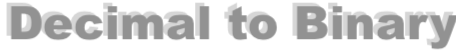How to Convert 57 to BinaryHere we will show you step-by-step how to convert the decimal number 57 to binary.

First, note that decimal numbers use 10 digits (0, 1, 2, 3, 4, 5, 6, 7, 8, and 9) and binary numbers use only 2 digits (0 and 1).

As we explain the steps to converting 57 to binary, it is important to know the name of the parts of a division problem. In a problem like A divided by B equals C, A is the Dividend, B is the Divisor and C is the Quotient.

The Quotient has two parts. The Whole part and the Fractional part. The Fractional part is also known as the Remainder.

Step 1) Divide 57 by 2 to get the Quotient. Keep the Whole part for the next step and set the Remainder aside.

Step 2) Divide the Whole part of the Quotient from Step 1 by 2. Again, keep the Whole part and set the Remainder aside.

Step 3) Repeat Step 2 above until the Whole part is 0.

Step 4) Write down the Remainders in reverse order to get the answer to 57 as a binary.

Here we will show our work so you can follow along:

57 / 2 = 28 with 1 remainder
28 / 2 = 14 with 0 remainder
14 / 2 = 7 with 0 remainder
7 / 2 = 3 with 1 remainder
3 / 2 = 1 with 1 remainder
1 / 2 = 0 with 1 remainder

Then, when we put the remainders together in reverse order, we get the answer. The decimal number 57 converted to binary is therefore:

111001

So what we did on the page was to Convert A10 to B2, where A is the decimal number 57 and B is the binary number 111001. Which means that you can display decimal number 57 to binary in mathematical terms as follows:

5710 = 1110012

Decimal to Binary Converter
Need another decimal number as a binary number? How to convert 57 to binary is not all we know. Convert another decimal to binary below:

How to Convert 58 to Binary
Here is our next decimal to binary tutorial on our list.